# Implode an array with jQuery/JavaScript

Given an array of elements and the task is to implode (join the elements of an array) the array elements.

Method 1: Using join() method: The join() method can be used to join an array with a separator and return it as a string. The join() method takes in the optional parameter of a separator. The separator is assumed to be a comma, if not specified. If the array contains only one string, then it would be returned without the separator.

Syntax:

`array.join(separator)`

Example:

 ` ` `<``html``> ` `     `  `<``head``> ` `    ``<``title``> ` `        ``Implode an array with jQuery/Javascript? ` `    `` ` ` ` ` `  `<``body``> ` `    ``<``h1` `style``=``"color: green"``> ` `        ``GeeksforGeeks ` `    `` ` `     `  `    ``<``b``> ` `        ``Implode an array with jQuery/Javascript?  ` `    `` ` `     `  `    ``<``p``> ` `        ``Original array is ["One", ` `        ``"Two", "Three", "Four", "Five"] ` `    `` ` `     `  `    ``<``p``> ` `        ``Imploded array is:  ` `        ``<``span` `class``=``"output"``> ` `    `` ` ` `  `    ``<``button` `onclick``=``"implodeArray()"``> ` `        ``Implode Array ` `    `` ` `     `  `    ``<``script` `type``=``"text/javascript"``> ` `     `  `        ``function implodeArray() { ` `            ``originalArray = ` `                ``["One", "Two", "Three", "Four", "Five"]; ` `         `  `            ``separator = ' '; ` `            ``implodedArray = originalArray.join(separator); ` ` `  `            ``console.log(implodedArray); ` `            ``document.querySelector('.output').textContent ` `                    ``= implodedArray; ` `        ``} ` `    `` ` ` ` ` `  `                     `

Output:

• Before clicking the button: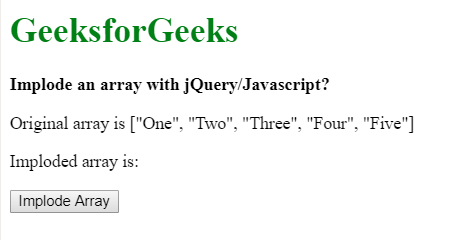• After clicking the button: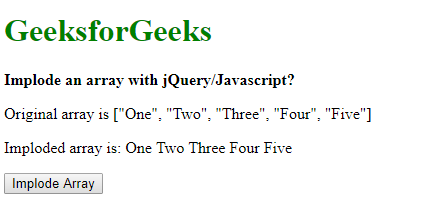• Console Output: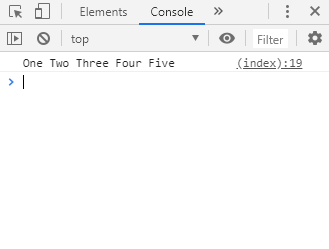Method 2: Iterating through the array and create a new string with all the strings and concatenated separators: The implode function can be created by looping through the array and concatenating it to one base string. The separator is added after concatenating each string unless it is the last string in the array. This prevents from adding the separator to the end of the last string in the array.
This method is slower than the join() method as a large number of strings are temporarily created during the concatenation of the base string.

Example:

 ` ` `<``html``> ` `     `  `<``head``> ` `    ``<``title``> ` `        ``Implode an array with jQuery/Javascript?  ` `    `` ` ` ` ` `  `<``body``> ` `    ``<``h1` `style``=``"color: green"``> ` `        ``GeeksforGeeks ` `    `` ` `     `  `    ``<``b``> ` `        ``Implode an array with jQuery/Javascript?  ` `    `` ` `     `  `    ``<``p``> ` `        ``Original array is ["One", ` `        ``"Two", "Three", "Four", "Five"] ` `    `` ` `     `  `    ``<``p``> ` `        ``Imploded array is:  ` `        ``<``span` `class``=``"output"``> ` `    `` ` ` `  `    ``<``button` `onclick``=``"implodeArray()"``> ` `        ``Implode Array ` `    `` ` `     `  `    ``<``script` `type``=``"text/javascript"``> ` `     `  `        ``function implodeArray() { ` `            ``originalArray = ` `            ``["One", "Two", "Three", "Four", "Five"]; ` `             `  `            ``separator = '+'; ` `            ``implodedArray = ''; ` ` `  `            ``for(let i = 0; i < ``originalArray.length``; i++) { ` ` `  `                ``// add a string from original array ` `                ``implodedArray += originalArray[i]; ` ` `  `                ``// unless the iterator reaches the end of ` `                ``// the array add the separator string ` `                ``if(i != originalArray.length - 1){ ` `                    ``implodedArray += separator;  ` `                ``} ` `            ``} ` ` `  `            ``console.log(implodedArray); ` `            ``document.querySelector('.output')``.textContent` `                    ``= ``implodedArray``; ` `        ``} ` `    `` ` ` ` ` `  `                     `

Output:

• Before clicking the button:• After clicking the button: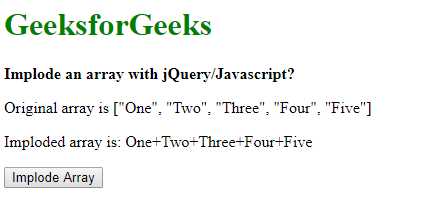• Console Output: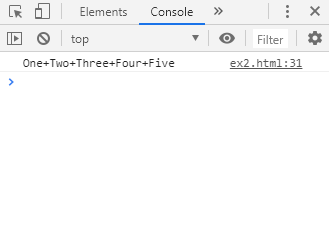My Personal Notes arrow_drop_upCheck out this Author's contributed articles.

If you like GeeksforGeeks and would like to contribute, you can also write an article using contribute.geeksforgeeks.org or mail your article to contribute@geeksforgeeks.org. See your article appearing on the GeeksforGeeks main page and help other Geeks.

Please Improve this article if you find anything incorrect by clicking on the "Improve Article" button below.